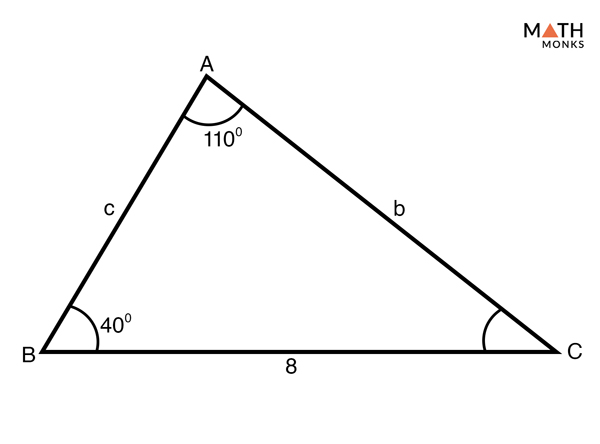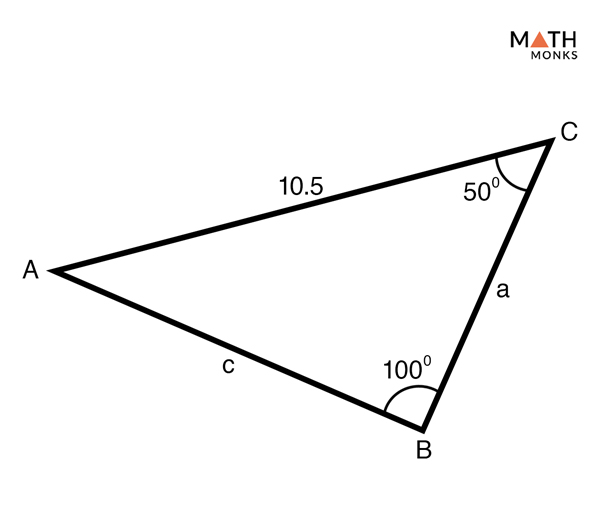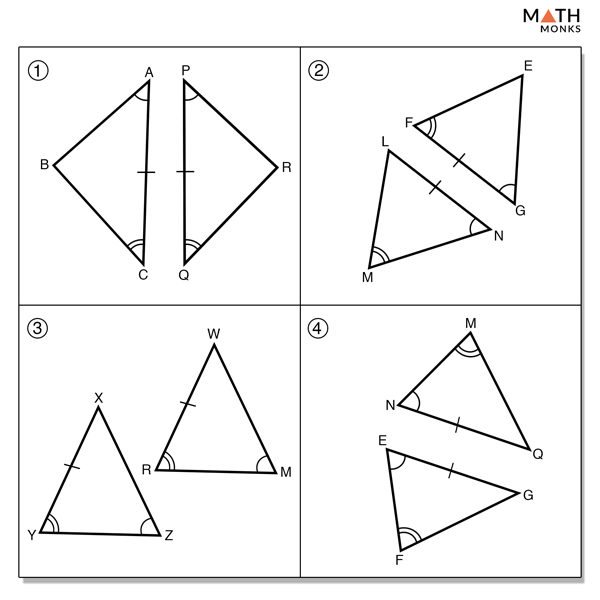# AAS Triangle

## What are AAS Triangles

AAS meaning ‘Angle-Angle-Side’ are triangles where two angles and any one of the non-included sides are known. Shown below is an AAS triangle, △ABC, with two known angles, ∠A and ∠B, and the known side length BC = ‘a’.

## Solving AAS Triangles

It involves two steps:

Step1: Use the angle sum rule of a triangle to find the missing angle

Step 2: Use the Law of Sines to find each of the other two sides

Let us solve some examples to understand the concept better.

### Solved ExamplesFind the missing sides and the angle in the given AAS triangle.

Solution:

In the triangle, the given angles and side is:
∠A = 110°
∠B = 40°
Side a = 8
Step 1:
Using the angle sum theorem, we will find the missing angle, ∠C
∠A + ∠B + ∠C = 180°, here ∠A = 110°, ∠B = 40°
110° + 40° + ∠C = 180°
∠C = 180° – (110° + 40°)
∠C = 30°
Step 2:
Now, we will find side b using the Law of Sines
a/sin A = b/sin B, here ∠A = 110°, a = 8, ∠B= 40°
8/ sin (110°) = b/ sin (40°)
b = sin (40°) x 8/ sin (110°)
b = 0.64 x 8/0.93
b = 5.50
Similarly, we will find side c using the Law of Sines
b/sin B = c/sin C, here b = 5.50, ∠B= 40°, ∠C = 30°
5.50/sin 40° = c/sin 30°
c = 5.50 x sin 30°/ sin 40°
c = 5.50 x 0.5/0.64
c = 4.29
This is to note as a rule that it is always better to use the sides and angles that are given to us rather than ones we have just worked out.Find the missing sides and the angle in the given triangle.

Solution:

The given triangle is also an AAS triangle having angles and side:
∠B = 100°
∠C = 50°
Side b = 10.5
Step 1:
Using the angle sum theorem, we will find the missing angle, ∠A
∠A + ∠B + ∠C = 180°, here ∠B = 100°, ∠C = 50°
∠A + 100° + 50° = 180°
∠A = 180° – (100° + 50°)
∠A = 30°
Step 2:
Now, we will find side c using the Law of Sines
b/sin B = c/sin C, here b = 10.5, ∠B = 100°, ∠C = 50°
10.5/sin 100° = c/sin 50°
c = 10.5 x sin 50°/ sin 100°
c = 10.5 x 0.76/0.98
c = 8.14
Similarly, we will find side a using the Law of Sines
a/sin A = b/sin B, here ∠A = 30°, b = 10.5, ∠B = 100°
a/sin 30° = 10.5/sin 100°
a = sin 30° x 10.5/ sin 100°
a = 0.5 x 10.5/0.98
a = 5.35

## AAS Triangle Congruence Theorem

Let us prove the above theorem.

### Prove AAS Triangle Congruence Theorem

To prove: △BCA ≅△DCA

Proof:Identify which pair of triangles below does NOT illustrate an angle angle side (AAS) relationship.

Solution:

Option (1) and (2)